Related Articles

# How to find distinct values of multiple columns in PySpark ?

• Last Updated : 04 Jul, 2021

In this article, we will discuss how to find distinct values of multiple columns in PySpark dataframe.

Let’s create a sample dataframe for demonstration:

## Python3

 `# importing module``import` `pyspark`` ` `# importing sparksession from pyspark.sql module``from` `pyspark.sql ``import` `SparkSession`` ` `# creating sparksession and giving an app name``spark ``=` `SparkSession.builder.appName(``'sparkdf'``).getOrCreate()`` ` `# list  of employee data``data ``=` `[[``"1"``, ``"Tezas"``, ``"Google"``],``        ``[``"2"``, ``"Mohit Rawat"``, ``"Rakuten"``],``        ``[``"3"``, ``"rohith"``, ``"Geeksforgeeks"``],``        ``[``"4"``, ``"Nancy"``, ``"IBM"``],``        ``[``"1"``, ``"Raghav"``, ``"Wipro"``],``        ``[``"4"``, ``"Komal"``, ``"Amazon"``]]`` ` `# specify column names``columns ``=` `[``'ID'``, ``'NAME'``, ``'Company'``]`` ` `# creating a dataframe from the lists of data``dataframe ``=` `spark.createDataFrame(data, columns)`` ` `dataframe.show()`

Output: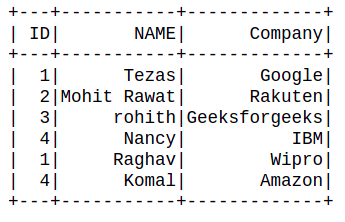Method 1: Using distinct() method

The distinct() method is utilized to drop/remove the duplicate elements from the DataFrame.

Syntax: df.distinct(column)

Example 1: Get a distinct Row of all Dataframe.

## Python3

 `dataframe.distinct().show()`

Output: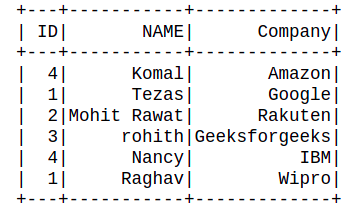Example 2: Get distinct Value of single Columns.

It can be done by passing a single column name with dataframe.

## Python3

 `dataframe.select(``'NAME'``).distinct().show()`

Output: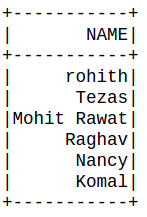Example 3: Get distinct Value of Multiple Columns.

It can be done by passing multiple column names as a form of a list with dataframe.

## Python3

 `dataframe.select(``'ID'``,``"NAME"``).distinct().show()`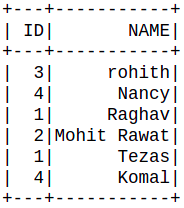Method 2: Using dropDuplicates() method.

The dropDuplicates() used to remove rows that have the same values on multiple selected columns.

Syntax: df.dropDuplicates()

Example 1: Get a distinct Row of all Dataframe.

## Python3

 `dataframe.dropDuplicates().show()`

Output:Example 2: Get distinct Value of single Columns.

It can be done by passing a single column name with dataframe.

## Python3

 `dataframe.select(``"NAME"``).dropDuplicates().show()`

Output:Example 3: Get distinct Value of multiple Columns.

It can be done by passing multiple column names as a form of a list with dataframe.

## Python3

 `dataframe.dropDuplicates([``"NAME"``,``"ID"``]).select([``"ID"``,``"NAME"``]).show()`

Output: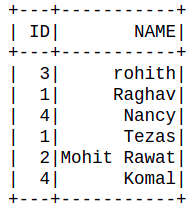Attention geek! Strengthen your foundations with the Python Programming Foundation Course and learn the basics.

To begin with, your interview preparations Enhance your Data Structures concepts with the Python DS Course. And to begin with your Machine Learning Journey, join the Machine Learning – Basic Level Course

My Personal Notes arrow_drop_up